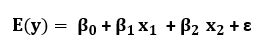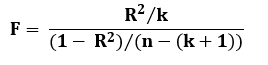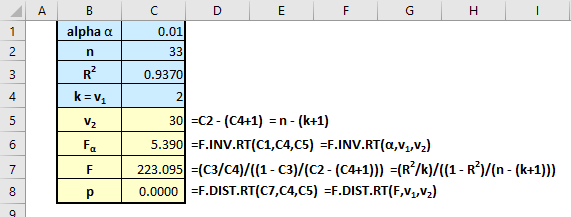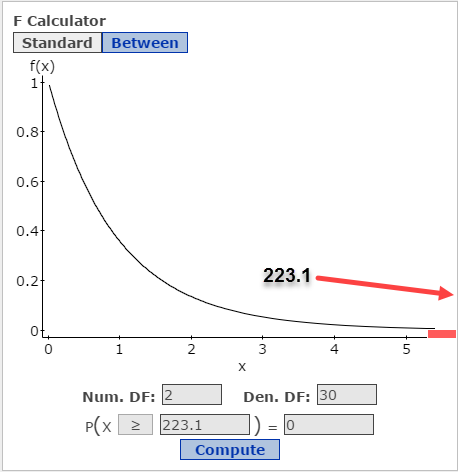# Perform Hypothesis Test for a Regression Model, Given R Squared

If you run into a problem, usually in an academic setting, where you only know the multiple coefficient of determination, R2, and are asked to test to see if the beta coefficients are non-zero, you can do this easily using Excel. You could also do it in StatCrunch using the Data > Compute tool, but I find it tedious compared to just building the solution in Excel. And, you can save the Excel solution for later reuse if you label and name it in a smart way.

Consider this problem:

Researchers want to use an analysis of social media to forecast the number of viewers, and thus ad revenues, for new TV series. They collected data on the pilot episodes for 33 series. The data included the number of times per minute a series was mentioned in the 24 hours after the pilots aired. It also included an analysis of the sentiment index of the mentions, i.e.  ratio of positive to negative mentions. R2 for the 1st order regression model they produced is 0.937; Ra2 = 0.933.

Test the model to see if it might be useful in forecasting ad revenues for a new TV series.

Solution:

• A first-order model consists of terms for quantitative independent variables. Because we have two independent variables, the model will be of the form:• Each β represents the slope of the line relating y to an x-term when all the other x-terms are held fixed. For example, if x1 is the mention rate, the β1 represents the change in revenue, y, for every 1-unit increase in the mention rate, holding the sentiment index, x2, constant.
• There are two x terms in the model, thus, k = 2.
• R2 is the multiple coefficient of determination. It represents the fraction of the variation in the dependent variable y explained by the regression equation, the least squares prediction.
• Ra2 provides an adjustment to R2, but includes consideration of the sample size and number of predictors in the model. The adjustment increases R2 only if an added predictor has a strong correlation to y and decreases R2 if the added predictor has a weak correlation to y. Generally, you should use the adjusted R2 to describe the predictive capability of the model. In this problem, I would report that the model explains 93.3% of the variation in y.
• The null hypothesis for regression models is that the slope coefficients are 0. Ho: β1 = β2 = 0.
• The alternative hypothesis is that at least on slope coefficient is non-zero. Ha: at least one βi ≠ 0.

The test statistic for this hypothesis test is F:• This global test for model usefulness is always a right-tail test, where the rejection region is F > Fα.
• The probability distribution for the F statistic is the F distribution. It is defined by two factors, the numerator degrees of freedom, v1, and the denominator degrees of freedom, v2. I discuss the f-distribution here.
• For our purposes in this problem, v1 = k and v2 = n-(k+1).

Here is the Excel solution:• Although you can use the Data > Compute tool in StatCrunch to calculate the F-statistics, I think using Excel for that purpose is easier. Once you have the F-statistic, we can use StatCrunch F calculator to double check and draw a sketch, though in this case, the F-statistic is so far to the right you cannot see it (note I added a bit of red color so you can see the rejection area).
• The first image shows StatCrunch found a right-tail critical value of F to be 5.39 for an alpha of 0.01. The second show the p-value for the test statistic is 0.• Because the p-values in both methods is essentially 0, the decision is to reject the null that all the slopes are zero. We can conclude at least one β is non-zero and the model is statistically useful.
• But, importantly, this does not mean the model is the best model – there may be another model that produces better estimates and predictions.
• If the global test indicates the model is useful, then tests of one or more of the individual β parameters could be performed. If you are given the results of these individual t-tests, interpret them in a similar fashion. See here for how to test individual betas using regression output.

This site uses Akismet to reduce spam. Learn how your comment data is processed.